Author: Oscar Cronquist Article last updated on February 22, 2023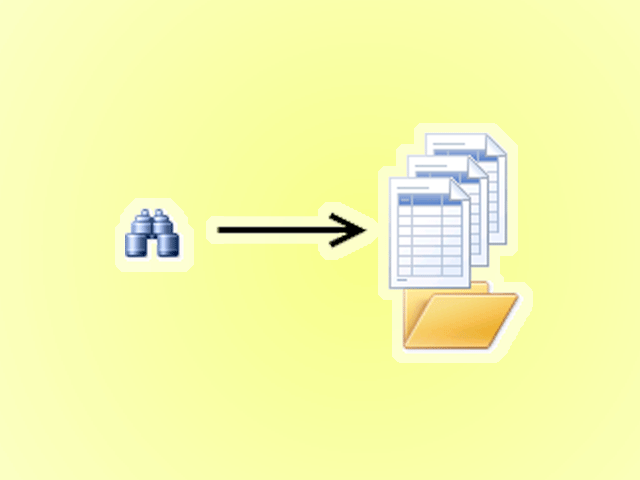Today I'll show you how to search all Excel workbooks with file extensions xls, xlsx and xlsm in a given folder for a specific string.

You can search workbooks with other file extensions as well, I will demonstrate what to change in the macro, later in this post.

The macro creates a new worksheet containing the search result. Each result contains a link to a cell where the search string was found.

You can then press with left mouse button on the link and Excel opens the corresponding workbook and locates the cell containing the search string.

There are also macros demonstrated here that allow you to search for multiple values and return a corresponding on the same row.

The following macro asks for a folder to search in and the search string.

### Search workbooks in folder for a single string

```'Name macro
Sub SearchWKBooks()

'Dimension variables and declare data types
Dim WS As Worksheet
Dim myfolder As String
Dim Str As String
Dim a As Single
Dim sht As Worksheet

'Create a new worksheet and svae it to object named WS

'Show dialog box and ask for a folder
With Application.FileDialog(msoFileDialogFolderPicker)
.Show

'Save selected folder path to variable myfolder
myfolder = .SelectedItems(1) & "\"
End With

'Show inputbox and ask for search string to use
Str = Application.InputBox(prompt:="Search string:", Title:="Search all workbooks in a folder", Type:=2)

'Stop macro if string is nothing
If Str = "" Then Exit Sub

'Save text "Search string:" to cell A1 on worksheet WS
WS.Range("A1") = "Search string:"

'Save text in variable Str to cell B1 on worksheet WS
WS.Range("B1") = Str

'Save text "Path:" to cell A2 on worksheet WS
WS.Range("A2") = "Path:"

'Save text in variable myfolder to cell B2 on worksheet WS
WS.Range("B2") = myfolder

'Save text "Workbook" to cell A3 on worksheet WS
WS.Range("A3") = "Workbook"

'Save text "Worksheet" to cell B3 on worksheet WS
WS.Range("B3") = "Worksheet"

'Save text "Cell Address" to cell C3 on worksheet WS

'Save text "Link" to cell D3 on worksheet WS

'Save 0 (zero) to variable a
a = 0

' The DIR function returns the first filename that matches the pathname specified in variable myfolder and save it to variable Value
Value = Dir(myfolder)

'Keep iterating until variable Value is nothing
Do Until Value = ""

'Check if Value is . or ..
If Value = "." Or Value = ".." Then

'If Value is not . or .. run these lines of code
Else

'Check If file names last three characters match xls or four last characters match xlsx or xlsm
If Right(Value, 3) = "xls" Or Right(Value, 4) = "xlsx" Or Right(Value, 4) = "xlsm" Then

'Enable error handling
On Error Resume Next

'Open workbook based on path in variable folder and file name in variable Value using password zzzzzzzzzzzz

'Check if an error has occured
If Err.Number > 0 Then

'Save path to column A based on counter variable a
WS.Range("A4").Offset(a, 0).Value = Value

'Save text "Password protected" to column B based on counter variable a

'Add 1 to the number stored in variable a
a = a + 1

'Continue here if no error is returned
Else

'Disable error handling
On Error GoTo 0

'Go through all worksheets in active workbook
For Each sht In ActiveWorkbook.Worksheets

'Seach worksheet based on value in variable Str and save result to object c
Set c = sht.Cells.Find(Str, LookIn:=xlValues, LookAt:=xlWhole, SearchOrder:=xlByRows, SearchDirection:=xlNext)

'Check if object c is not nothing
If Not c Is Nothing Then

'Repet following lines between Do and Loop
Do

'Save file name to column A and row number based on variable a
WS.Range("A4").Offset(a, 0).Value = Value

'Save worksheet name to column B and row number based on variable a
WS.Range("B4").Offset(a, 0).Value = sht.Name

'Save cell address to column C and row number based on variable a

'Increase count variable a with 1
a = a + 1

'Find next cell that matches and save to object c
Set c = sht.Cells.FindNext(c)

'Keep iterating until c is nothing and c is not equal to the first found cell in the same worksheet
End If

'Continue with next worksheet
Next sht
End If

'Close workbook and do not save any changes made
Workbooks(Value).Close False

'Diable error handling
On Error GoTo 0
End If
End If

'Find next file
Value = Dir

'Keep iterating
Loop

'Change cell widths to fit text
Cells.EntireColumn.AutoFit
End Sub
```

### Explaining the macro

The macro loops through all files in the given folder and if the file extension matches xls, xlsx or xlsm the workbook is opened.

If the workbook is password protected the macro outputs "Password protected" for that workbook.

If it successfully opens a workbook another loop is started that searches each worksheet in the workbook for the given search string.

### Where to put the code?

1. Copy macro (CTRL + c)
2. Go to the VB Editor (Alt + F11)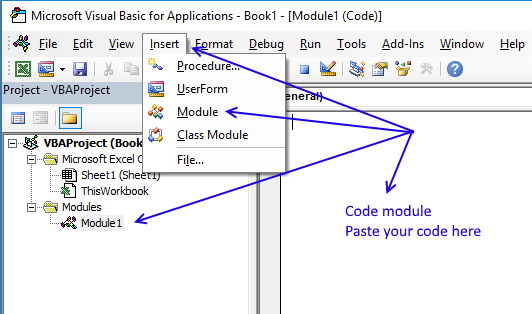3. Press with left mouse button on "Insert" on the top menu.
4. Press with left mouse button on "Module" to insert a code module to your workbook.
5. Paste code to the module. (CTRL + v)
7. Save your workbook as a macro-enabled workbook (*.xlsm file).
If you don't the macro will be gone the next time you open the same workbook.

### Example

Example, I have 5 workbooks in folder c:\temp\ containing many random numbers. The macro asks for the path and the search string.

It then creates this sheet.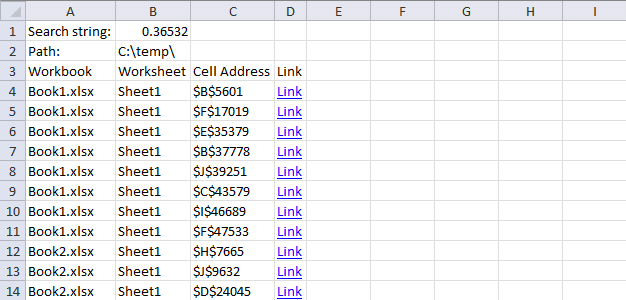Press with left mouse button on a link and excel loads the corresponding workbook and navigates to the specific cell.

### Search other file extensions

Change the following line to whatever file extensions you want to look for.

```If Right(Value, 3) = "xls" Or Right(Value, 4) = "xlsx" Or Right(Value, 4) = "xlsm" Then
```

### Get the Excel fileSearch-multiple-workbooks-in-a-folder.xlsm

### Search multiple strings in all workbooks in a folder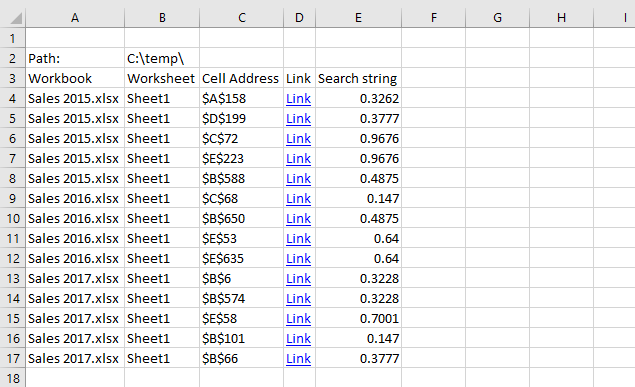The following macro allows you to search all workbooks in a folder for multiple search strings.

```'Name macro
Sub SearchWKBooks()

'Dimension variables and declare their data types
Dim WS As Worksheet
Dim myfolder As String
Dim Str As String
Dim a As Single
Dim sht As Worksheet
Dim RNG As Range

'Enable error handling
On Error Resume Next

'Show dialog box and ask for a cell range
Set RNG = Application.InputBox(Prompt:="Select a cell range containing search strings" _
, Title:="Select a range", Default:=ActiveCell.Address, Type:=8)

'Disable error handling
On Error GoTo 0

'Show dialog box and ask for a folder
With Application.FileDialog(msoFileDialogFolderPicker)
.Show
myfolder = .SelectedItems(1) & "\"
End With

'Write "Path:" to cell A2
WS.Range("A2") = "Path:"

'Write contents of variable myfolder to cell B2
WS.Range("B2") = myfolder

'Write "Workbook" to cell A3
WS.Range("A3") = "Workbook"

'Write "Worksheet" to cell B3
WS.Range("B3") = "Worksheet"

'Write "Cell Address" to cell C3

'Write "Search string" to cell E3
WS.Range("E3") = "Search string"

'Save 0 (zero) to variable a
a = 0

'The DIR function returns the first filename that matches the pathname specified in variable myfolder and saves it to variable Value
Value = Dir(myfolder)

'Keep iterating through all files and folders until variable Value is empty
Do Until Value = ""

'Check if Value is . meaning current directory or .. meaning parent directory
If Value = "." Or Value = ".." Then

'Go here if Value is not . or ..
Else

'Check if last three characters match xls or last four characters match xlsx or xlsm
If Right(Value, 3) = "xls" Or Right(Value, 4) = "xlsx" Or Right(Value, 4) = "xlsm" Then

'Enable error handling
On Error Resume Next

'Check if an error has occurred
If Err.Number > 0 Then

'Write contents of variable Value to cell A4 offset by number in variable a
WS.Range("A4").Offset(a, 0).Value = Value

'Write "Password protected" of variable Value to cell A4 offset by number in variable a

'Add 1 to number stored in variable a
a = a + 1
Else

'Disbale error handling
On Error GoTo 0

'Iterate through each worksheet in active workbook
For Each sht In ActiveWorkbook.Worksheets

'Iterate through all cells in variable RNG
For Each d In RNG

'Find cells in worksheet sht equal to value in cell d and save to range object variable c
Set c = sht.Cells.Find(d, LookIn:=xlValues, LookAt:=xlWhole, SearchOrder:=xlByRows, SearchDirection:=xlNext)

'Check if range object variable c is populated
If Not c Is Nothing Then

'Do ... Loop through all cells in range object variable c
Do

'Save contents of variable Value to cell A4 offset by variable a
WS.Range("A4").Offset(a, 0).Value = Value

'Save worksheet name to cell B4 offset by variable a
WS.Range("B4").Offset(a, 0).Value = sht.Name

'Save cell address to cell C4 offset by variable a

'Create a hyperlink in cell D4

'Save cell value in variable d to column E and row based on count variable a
WS.Range("E4").Offset(a, 0).Value = d

a = a + 1

'Go to next cell in range object variable c
Set c = sht.Cells.FindNext(c)

'Continue to loop as long as c is populated AND c is not equal to the first address stored in variable
End If
Next d
Next sht
End If
Workbooks(Value).Close False
On Error GoTo 0
End If
End If
Value = Dir
Loop
Cells.EntireColumn.AutoFit
End Sub
```

### How to use the macro

The macro asks for a cell range containing the search strings you want to look for. The next step is which folder to look in?

A new sheet is inserted and populated with data if values are found.

### Get the Excel fileSearch-multiple-workbooks-in-a-folderv2.xlsm

### Search multiple strings and return corresponding value(s) on the same row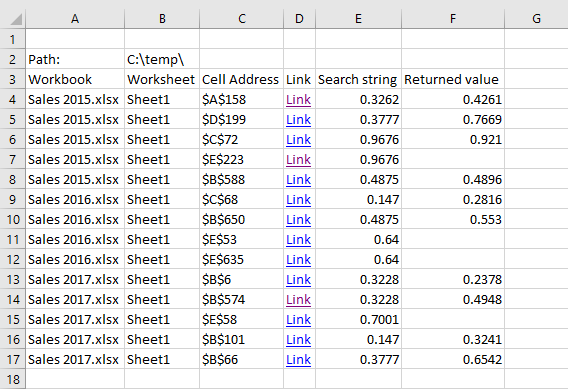The macro demonstrated above looks for multiple search strings in all Excel workbooks in a folder and also returns a value on the same row.

```Sub SearchWKBooks()
Dim WS As Worksheet
Dim myfolder As String
Dim Str As String
Dim a As Single
Dim sht As Worksheet
Dim RNG As Range

On Error Resume Next
'Show inputbox and ask for cell range containing search values
Set RNG = Application.InputBox(prompt:="Select a cell range containing search strings" _
, Title:="Select a range", Default:=ActiveCell.Address, Type:=8)

On Error GoTo 0

'Show inputbox and ask for cell offset
Str = Application.InputBox(prompt:="Cell Offset:", Title:="Offset", Type:=2)

With Application.FileDialog(msoFileDialogFolderPicker)
.Show
myfolder = .SelectedItems(1) & "\"
End With

WS.Range("A2") = "Path:"
WS.Range("B2") = myfolder
WS.Range("A3") = "Workbook"
WS.Range("B3") = "Worksheet"
WS.Range("E3") = "Search string"
WS.Range("F3") = "Returned value"

a = 0

Value = Dir(myfolder)
Do Until Value = ""
If Value = "." Or Value = ".." Then
Else
If Right(Value, 3) = "xls" Or Right(Value, 4) = "xlsx" Or Right(Value, 4) = "xlsm" Then
On Error Resume Next
If Err.Number > 0 Then
WS.Range("A4").Offset(a, 0).Value = Value
a = a + 1
Else
On Error GoTo 0
For Each sht In ActiveWorkbook.Worksheets
For Each d In RNG
'MsgBox d
Set c = sht.Cells.Find(d, LookIn:=xlValues, LookAt:=xlWhole, SearchOrder:=xlByRows, SearchDirection:=xlNext)
If Not c Is Nothing Then
Do
WS.Range("A4").Offset(a, 0).Value = Value
WS.Range("B4").Offset(a, 0).Value = sht.Name
WS.Range("E4").Offset(a, 0).Value = d

'Save value from cell based on offset variable Str
WS.Range("F4").Offset(a, 0).Value = c.Offset(0, Str).Value
a = a + 1
Set c = sht.Cells.FindNext(c)
End If
Next d
Next sht
End If
Workbooks(Value).Close False
On Error GoTo 0
End If
End If
Value = Dir
Loop
Cells.EntireColumn.AutoFit
End Sub

```

### How to use

1. Start macro (Alt+F8)
2. Select the cell range containing search strings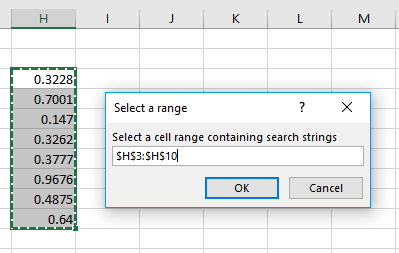3. Press with left mouse button on OK button.
4. Select how many cells to the right (positive numbers) or to the left (negative numbers) from the found value.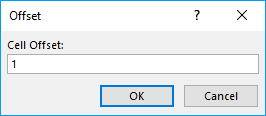Example, 1 will return the adjacent value to the right.
Example, -2 will return the value from a cell two columns to the left of the found value.
5. Select the folder you want to use.
6. Press with left mouse button on OK

The macro will now add a worksheet to your workbook and populate it with data if values are found.### Get the Excel fileSearch-multiple-workbooks-in-a-folder-return-value.xlsm

The following article explains how to search a folder and subfolders:

Recommended articles

Search all workbooks in a folder and sub folders – VBA
This article demonstrates macros that allow you to search for a text string(s) in multiple worksheets and workbooks located in […]